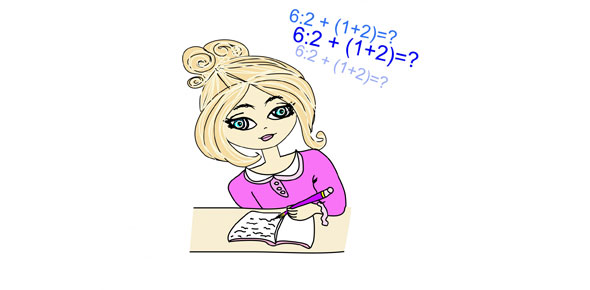# SAT Practice Math Questions For Mentors

14 Questions | Total Attempts: 16SettingsSAT Math practice questions

Related Topics
• 1.
A number is given as 3513N, where N is an positive integer that fills in the units digit. Which of the following values of N would result in 3513N being a multiple of 9?
• A.

3

• B.

4

• C.

5

• D.

6

• E.

7

• 2.
Solve for x: 2x + 3 = 4x - 3
• A.

0

• B.

1

• C.

3

• D.

5

• E.

7

• 3.
In the context of whole numbers, which of the following is not a true statement?
• A.

Every even number is divisible by two

• B.

Every number divisible by two is an even number

• C.

Every number not divisible by two is not odd

• D.

Every number that is odd is not divisible by two

• E.

Every number not divisible by two is composite

• 4.
(5 * 5 + 3) / 2 + 7 / 7 = ?
• A.

1

• B.

7

• C.

14

• D.

15

• E.

35

• 5.
A set of integers includes: 44, 66, 88, and x. If the mean of the scores is 80, what is x?
• A.

12

• B.

104

• C.

120

• D.

122

• E.

200

• 6.
John is older than Maria. Maria is younger than Sue. Terry is the oldest of the four of them. Who is the youngest?
• A.

John

• B.

Maria

• C.

Sue

• D.

Terry

• E.

They are all the same age

• 7.
If the length of the sides of a cube is tripled, by what factor is the volume of the cube increased?
• A.

3

• B.

6

• C.

9

• D.

15

• E.

27

• 8.
A fair coin is flipped three times. What is the chance that no head will appear?
• A.

1/8

• B.

1/4

• C.

1/3

• D.

1/2

• E.

0

• 9.
If a block has dimensions of 3, 4, and 5 units, how many 2 by 2 by 3 blocks can fit in the block?
• A.

4

• B.

5

• C.

8

• D.

12

• E.

15

• 10.
A six-sided dice is rolled twice. What is the chance that the sum of the two rolls will be exactly 10?
• A.

1/12

• B.

1/16

• C.

3/7

• D.

1/6

• E.

3/8

• 11.
In a class election between Ricky, Susie, and Mikey, Ricky earns 20% of the vote and Mikey earns 30% of the vote. There are 24 members of the class. How many votes did Susie receive?
• A.

4

• B.

8

• C.

12

• D.

16

• E.

20

• 12.
The letters of the word: "alphabets" are placed into a hat and scrambled. What is the probability of choosing a vowel at random from the hat?
• A.

1/12

• B.

1/6

• C.

1/3

• D.

2/9

• E.

4/9

• 13.
If Mike can buy four apples for one pear and four pears for one peach, and if one peach is equal to eight bananas, how many bananas will Mike receive for two apples?
• A.

1

• B.

2

• C.

4

• D.

6

• E.

8

• 14.
If Pete can run five miles in one hour, how many hours would it take him to run 25 miles?
• A.

3

• B.

4

• C.

5

• D.

10

• E.

15